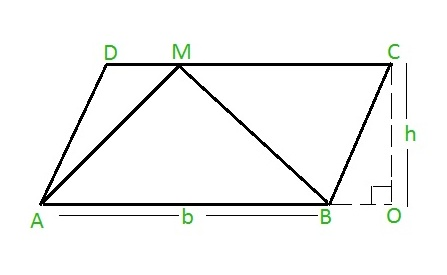# Area of a triangle inside a parallelogram

Given the base and height of the parallelogram ABCD are b and h respectively. The task is to calculate the area of the triangle ▲ABM (M can be any point on upper side) constructed on the base AB of the parallelogram as shown below:Examples:

```Input: b = 30, h = 40
Output: 600.000000
```

Approach:

Area of a triangle constructed on the base of parallelogram and touching at any point on the opposite parallel side of the parallelogram can be given as = 0.5 * base * height

Hence, Area of ▲ABM = 0.5 * b * h

Below is the implementation of the above approach:

## C++

 `#include ` `using` `namespace` `std; ` ` `  `// function to calculate the area ` `float` `CalArea(``float` `b, ``float` `h) ` `{ ` `    ``return` `(0.5 * b * h); ` `} ` `// driver code ` `int` `main() ` `{ ` `    ``float` `b, h, Area; ` `    ``b = 30; ` `    ``h = 40; ` ` `  `    ``// function calling ` `    ``Area = CalArea(b, h); ` `    ``// displaying the area ` `    ``cout << ``"Area of Triangle is :"` `<< Area; ` `    ``return` `0; ` `} `

## C

 `#include ` ` `  `// function to calculate the area ` `float` `CalArea(``float` `b, ``float` `h) ` `{ ` `    ``return` `(0.5 * b * h); ` `} ` ` `  `// driver code ` `int` `main() ` `{ ` `    ``float` `b, h, Area; ` `    ``b = 30; ` `    ``h = 40; ` ` `  `    ``// function calling ` `    ``Area = CalArea(b, h); ` ` `  `    ``// displaying the area ` `    ``printf``(``"Area of Triangle is : %f\n"``, Area); ` `    ``return` `0; ` `} `

## Java

 `public` `class` `parallelogram { ` `    ``public` `static` `void` `main(String args[]) ` `    ``{ ` `        ``double` `b = ``30``; ` `        ``double` `h = ``40``; ` ` `  `        ``// formula for calculating the area ` `        ``double` `area_triangle = ``0.5` `* b * h; ` ` `  `        ``// displaying the area ` `        ``System.out.println(``"Area of the Triangle = "` `+ area_triangle); ` `    ``} ` `} `

## Python

 `b ``=` `30`  `h ``=` `40`   ` `  `# formula for finding the area ` `area_triangle ``=` `0.5` `*` `b ``*` `h ` ` `  `# displaying the output ` `print``(``"Area of the triangle = "``+``str``(area_triangle)) `

## C#

 `using` `System; ` `class` `parallelogram { ` `    ``public` `static` `void` `Main() ` `    ``{ ` `        ``double` `b = 30; ` `        ``double` `h = 40; ` ` `  `        ``// formula for calculating the area ` `        ``double` `area_triangle = 0.5 * b * h; ` ` `  `        ``// displaying the area ` `        ``Console.WriteLine(``"Area of the triangle = "` `+ area_triangle); ` `    ``} ` `} `

## PHP

 `  `

Output:

```Area of triangle is : 600.000000
```

My Personal Notes arrow_drop_upCheck out this Author's contributed articles.

If you like GeeksforGeeks and would like to contribute, you can also write an article using contribute.geeksforgeeks.org or mail your article to contribute@geeksforgeeks.org. See your article appearing on the GeeksforGeeks main page and help other Geeks.

Please Improve this article if you find anything incorrect by clicking on the "Improve Article" button below.